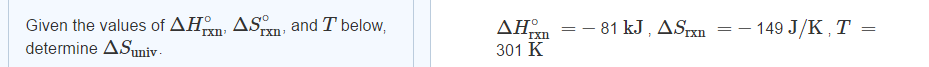# Problem: Given the values of ΔHºrxn, ΔSºrxn, and T below determine ΔSuniv.ΔHºrxn = -81 kJ,ΔSrxn = -149 J/K,T = 301 ºKExpress your answer using two significant figures.

###### FREE Expert Solution
89% (222 ratings)###### Problem Details

Given the values of ΔHºrxn, ΔSºrxn, and T below determine ΔSuniv.
ΔHºrxn = -81 kJ,
ΔSrxn = -149 J/K,
T = 301 ºK